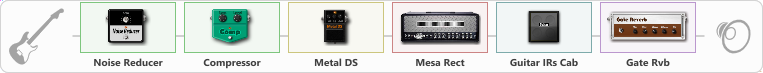Discussion in 'ToneLib-GFX presets' started by AmegoO, May 26, 2021.

Effects chain:Effect: "Noise Reducer" (Динамика / Фильтр), active - "yes"
{
"Sens" = 78
"Mode" = Hard
}

Effect: "Compressor" (Динамика / Фильтр), active - "yes"
{
"Sense" = 21
"Level" = 50
}

Effect: "Metal DS" (Перегрузка / Искажение), active - "yes"
{
"Dist" = 100
"Bass" = 74
"Middle" = 60
"Treble" = 46
"Level" = 57
}

Effect: "Mesa Rect" (Усилители симуляторы), active - "yes"
{
"Gain" = 50
"Bass" = 50
"Middle" = 50
"Treble" = 50
"Presence" = 50
"Master" = 50
"Level (dB)" = 0
}

Effect: "Guitar IRs Cab" (Кабинеты), active - "yes"
{
"Model" = Mesa Rectifier (4x12")
"Mic Position" = Middle
"Mic Distance" = Near
"Low Cut (Hz)" = 60
"Hi Cut (kHz)" = 20.0
"Mix" = 100
"Level (dB)" = 0
}

Effect: "Gate Rvb" (Реверберация), active - "yes"
{
"Time" = 97
"PreDelay" = 0
"LoDamp" = 30
"HiDamp" = 25
"Type" = Convent
"Mix" = 28
}

Note: You will need to download and install the ToneLib-GFX software to use the preset.